×#### Thank you for registering.

One of our academic counsellors will contact you within 1 working day.

Click to Chat

1800-1023-196

+91-120-4616500

CART 0

• 0

MY CART (5)

Use Coupon: CART20 and get 20% off on all online Study Material

ITEM
DETAILS
MRP
DISCOUNT
FINAL PRICE
Total Price: Rs.

There are no items in this cart.
Continue Shopping• Complete JEE Main/Advanced Course and Test Series
• OFFERED PRICE: Rs. 15,900
• View Details

```Chapter 14: Compound Interest Exercise – 14.3

Question: 1

On what sum will the compound interest at 5% per annum for 2 years compounded annually be Rs 164?

Solution: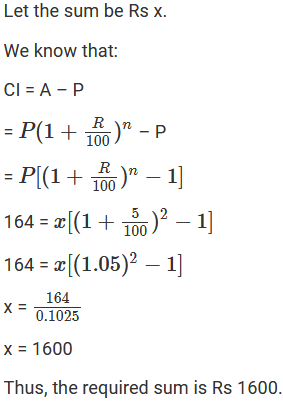Question: 2

Find the principal if the interest compounded annually at the rate of 10% for two years is Rs 210.

Solution: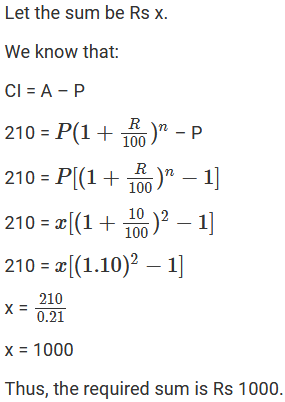Question: 3

A sum amounts to Rs 756.25 at 10% per annum in 2 years, compounded annually. Find the sum.

Solution: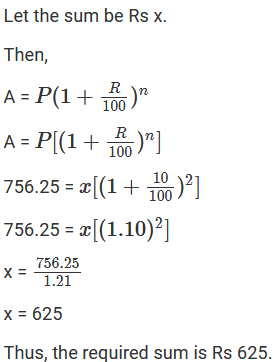Question: 4

What sum will amount to Rs 4913 in 18 months, if the rate of interest is 12(1/2)% per annum, compounded half-yearly?

Solution:Question: 5

The difference between the compound interest and simple interest on a certain sum at 15% per annum for 3 years is Rs 283.50. Find the sum.

Solution:Question: 6

Rachana borrowed a certain sum at the rate of 15% per annum. If she paid at the end of two years Rs 1290 as interest compounded annually, find the sum she borrowed.

Solution:Question: 7

The interest on a sum of Rs 2000 is being compounded annually at the rate of 4% per annum. Find the period for which the compound interest is Rs 163.20.

Solution: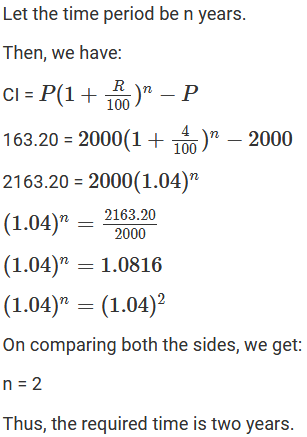Question: 8

In how much time would Rs 5000 amount to Rs 6655 at 10% per annum compound interest?

Solution:Question: 9

In what time will Rs 4400 become Rs 4576 at 8% per annum interest compounded half-yearly?

Solution:Question: 10

The difference between the S.I. and C.I. on a certain sum of money for 2 years at 4% per annum is Rs 20. Find the sum.

Solution: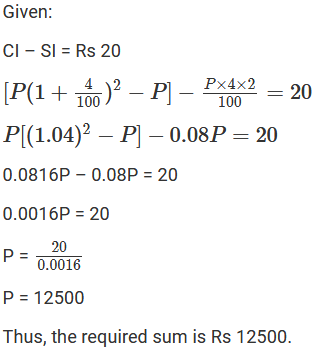Question: 11

In what time will Rs 1000 amount to Rs 1331 at 10% per annum, compound interest?

Solution: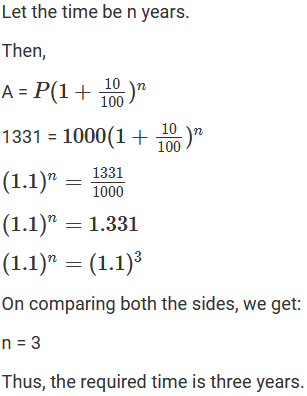Question: 12

At what rate percent compound interest per annum will Rs 640 amount to Rs 774.40 in 2 years?

Solution:Question: 13

Find the rate percent per annum if Rs 2000 amount to Rs 2662 in 1(1/2) years, interest being compounded half-yearly?

Solution: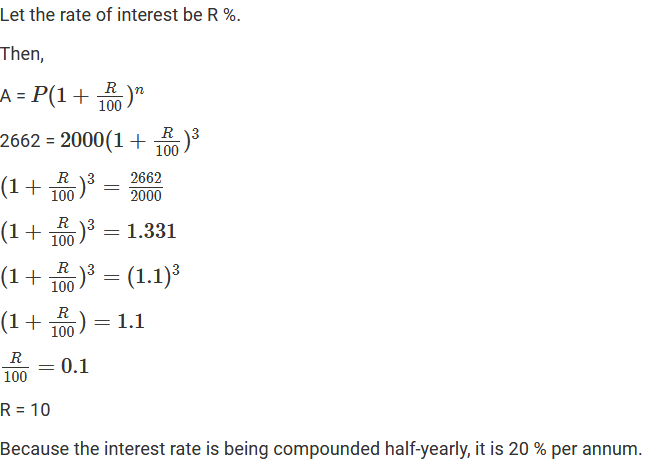Question: 14

Kamala borrowed from Ratan a certain sum at a certain rate for two years simple interest. She lent this sum at the same rate to Hari for two years compound interest. At the end of two years, she received Rs 210 as compound interest but paid Rs 200 only as simple interest. Find the sum and the rate of interest.

Solution:Question: 15

Find the rate percent per annum, if Rs 2000 amount to Rs 2315.25 in a year and a half, interest being compounded six monthly.

Solution:Question: 16

Find the rate at which a sum of money will double Itself in 3 years if the interest is compounded annually.

Solution:Question: 17

Find the rate at which a sum of money will become four times the original amount in 2 years if the interest is compounded half-yearly.

Solution:Question: 18

A certain sum amounts to Rs 6832 in 2 years at 8% compounded interest. Find the sum.

Solution: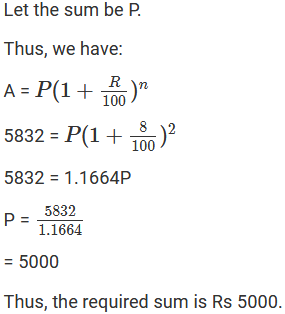Question: 19

The difference between the compound interest and simple interest on a certain sum for 2 years at 7.5% per annum is Rs 360. Find the sum.

Solution: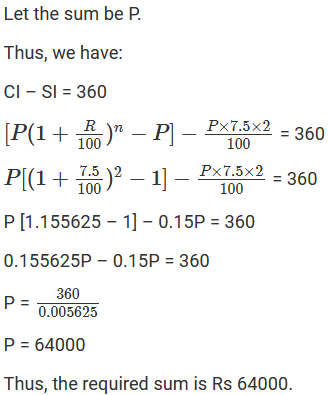Question: 20

The difference in simple interest and compound interest on a certain sum of money at 6(2/3)% per annum for 3 years is Rs 46. Determine the sum.

Solution: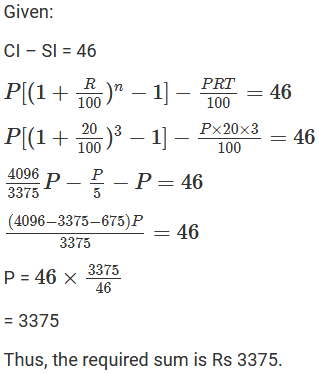Question: 21

Ishita invested a sum of Rs 12000 at 5% per annum compound interest. She received an amount of Rs 13230 after years. Find the value of n.

Solution: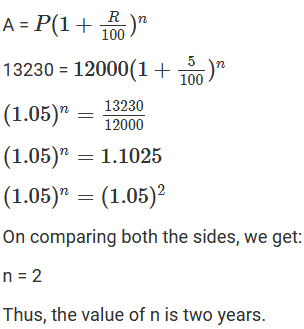Question: 22

At what rate percent per annum will a sum of Rs 4000 yield compound interest of Rs 410 in 2 years?

Solution: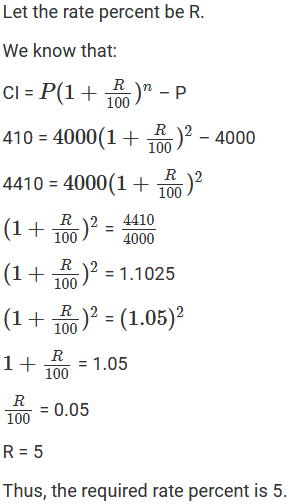Question: 23

A sum of money deposited at 2% per annum compounded annually becomes Rs 10404 at the end of 2 years. Find the sum deposited.

Solution: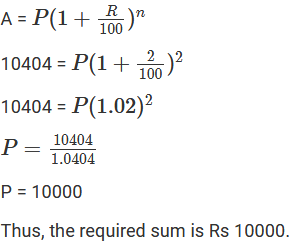Question: 24

In how much time will a sum of Rs 1600 amount to Rs 1852.20 at 5% per annum compound interest?

Solution: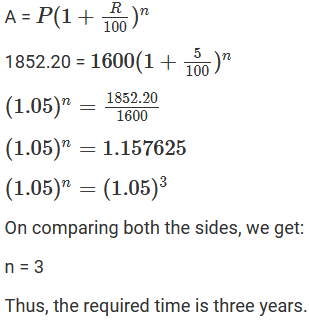Question: 25

At what rate percent will a sum of Rs 1000 amount to Rs 1102.50 in 2 years at compound interest?

Solution:Question: 26

The compound interest on Rs 1800 at 10% per annum for a certain period of time is Rs 378. Find the time in years.

Solution: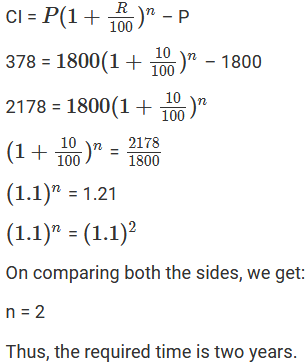Question: 27

What sum of money will amount to Rs 45582.25 at 6(3/4)% per annum in two years, interest being compounded annually?

Solution:Question: 28

Sum of money amounts to. Rs 453690 in 2 years at 6.5% per annum compounded annually. Find the sum.

Solution: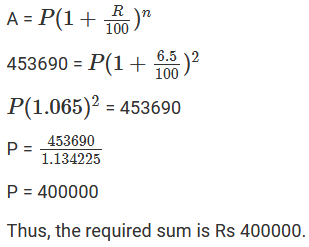```### Course Features

• 728 Video Lectures
• Revision Notes
• Previous Year Papers
• Mind Map
• Study Planner
• NCERT Solutions
• Discussion Forum
• Test paper with Video Solution